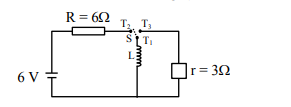# Consider an electrical circuit containing a two way switch ' S '.Question:

Consider an electrical circuit containing a two way switch ' $S$ '. Initially $S$ is open and then $T_{1}$ is connected to $\mathrm{T}_{2}$. As the current in $\mathrm{R}=6 \Omega$ attains a maximum value of steady state level, $T_{1}$ is disconnected from $\mathrm{T}_{2}$ and immediately connected to $\mathrm{T}_{3}$. Potential drop across $\mathrm{r}=3 \Omega$ resistor

immediately after $T_{1}$ is connected to $T_{3}$ is__________ $\mathrm{V} .$

(Round off to the Nearest Integer)Solution:

When $T_{1}$ and $T_{2}$ are connected, then the steady

state current in the inductor $\mathrm{I}=\frac{6}{6}=1 \mathrm{~A}$

When $T_{1}$ and $T_{3}$ are connected then curren through inductor remains same. So potentia difference across $3 \Omega$

$\mathrm{V}=\mathrm{Ir}=1 \times 3=3$ volt